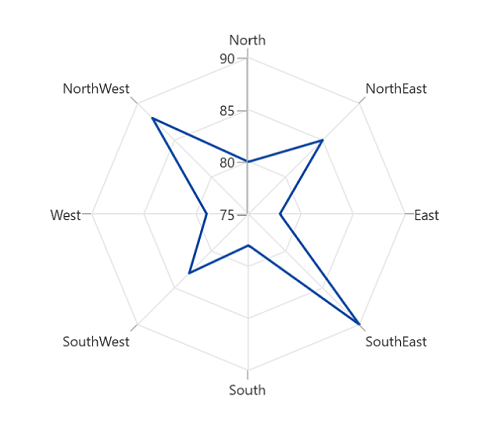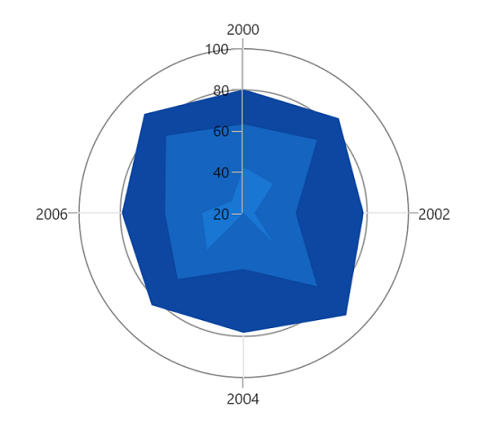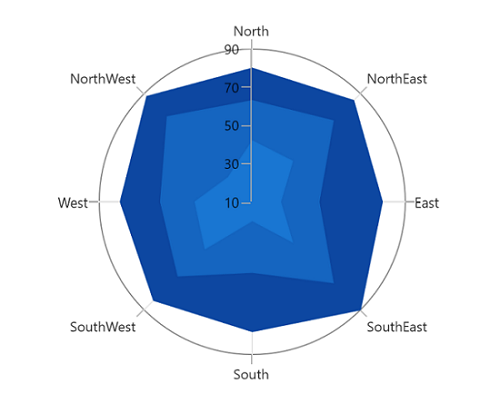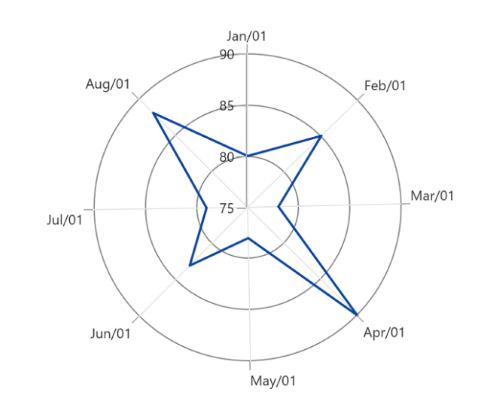# Types of Axis in WinUI Chart (SfPolarChart)

21 Nov 20224 minutes to read

Polar chart supports the following types of chart axis.

• CategoryAxis
• NumericalAxis
• DateTimeAxis

## Category Axis

The CategoryAxis is an indexed based axis that plots values based on the index of the data point collection. The points are equally spaced here.

``````<chart:SfPolarChart>

<chart:SfPolarChart.PrimaryAxis>
<chart:CategoryAxis/>
</chart:SfPolarChart.PrimaryAxis>

<chart:SfPolarChart.SecondaryAxis>
<chart:NumericalAxis/>
</chart:SfPolarChart.SecondaryAxis>
...

</chart:SfPolarChart>``````
``````SfPolarChart chart = new SfPolarChart();
chart.PrimaryAxis = new CategoryAxis();
chart.SecondaryAxis = new NumericalAxis();
...``````## Numerical Axis

The NumericalAxis is used to plot the numerical values to the chart. NumericalAxis can be defined for both PrimaryAxis and SecondaryAxis.

``````<chart:SfPolarChart>

<chart:SfPolarChart.PrimaryAxis>
<chart:NumericalAxis/>
</chart:SfPolarChart.PrimaryAxis>

<chart:SfPolarChart.SecondaryAxis>
<chart:NumericalAxis/>
</chart:SfPolarChart.SecondaryAxis>
...

</chart:SfPolarChart>``````
``````SfPolarChart chart = new SfPolarChart();
...
chart.PrimaryAxis = new NumericalAxis();
chart.SecondaryAxis = new NumericalAxis();
...``````### Customizing the range

Maximum and Minimum properties of axis is used for setting the maximum and minimum value of the axis range respectively.

NOTE

If minimum or maximum value is set, the other value is calculated by default internally.

``````<chart:SfPolarChart>
<chart:SfPolarChart.SecondaryAxis>
<chart:NumericalAxis Minimum="10"
Maximum="90"
Interval="20" />
</chart:SfPolarChart.SecondaryAxis>
...
</chart:SfPolarChart>``````
``````SfPolarChart chart = new SfPolarChart();
...
chart.SecondaryAxis = new NumericalAxis()
{
Maximum = 90,
Minimum = 10,
Interval = 20,
};
...``````## DateTime Axis

The DateTimeAxis is used to plot the chart with `DateTime` values.

``````<chart:SfPolarChart>

<chart:SfPolarChart.PrimaryAxis>
<chart:DateTimeAxis Interval="1"
IntervalType="Months">
<chart:DateTimeAxis.LabelStyle>
<chart:LabelStyle LabelFormat="MMM/dd" />
</chart:DateTimeAxis.LabelStyle>
</chart:DateTimeAxis>
</chart:SfPolarChart.PrimaryAxis>

<chart:SfPolarChart.SecondaryAxis>
<chart:NumericalAxis/>
</chart:SfPolarChart.SecondaryAxis>
...

</chart:SfPolarChart>``````
``````SfPolarChart chart = new SfPolarChart();

chart.PrimaryAxis = new DateTimeAxis()
{
Interval = 1,
IntervalType = DateTimeIntervalType.Months,
LabelStyle = new LabelStyle() { LabelFormat="MMM/dd" },
};

chart.SecondaryAxis = new NumericalAxis();
...``````## Events

### ActualRangeChanged

The ActualRangeChanged event is triggered when the actual range of the axis is changed. The argument contains the following information:

• ActualMinimum - used to get the actual minimum value of the axis.
• ActualMaximum - used to get the actual maximum value of the axis.

### LabelCreated

The LabelCreated event is triggered when the axis label is created. The argument contains the following information:

• Label - Used to get or set the text of axis label.
• Position - Used to get the position of label.
• LabelStyle - Used to customize the appearance of axis labels.